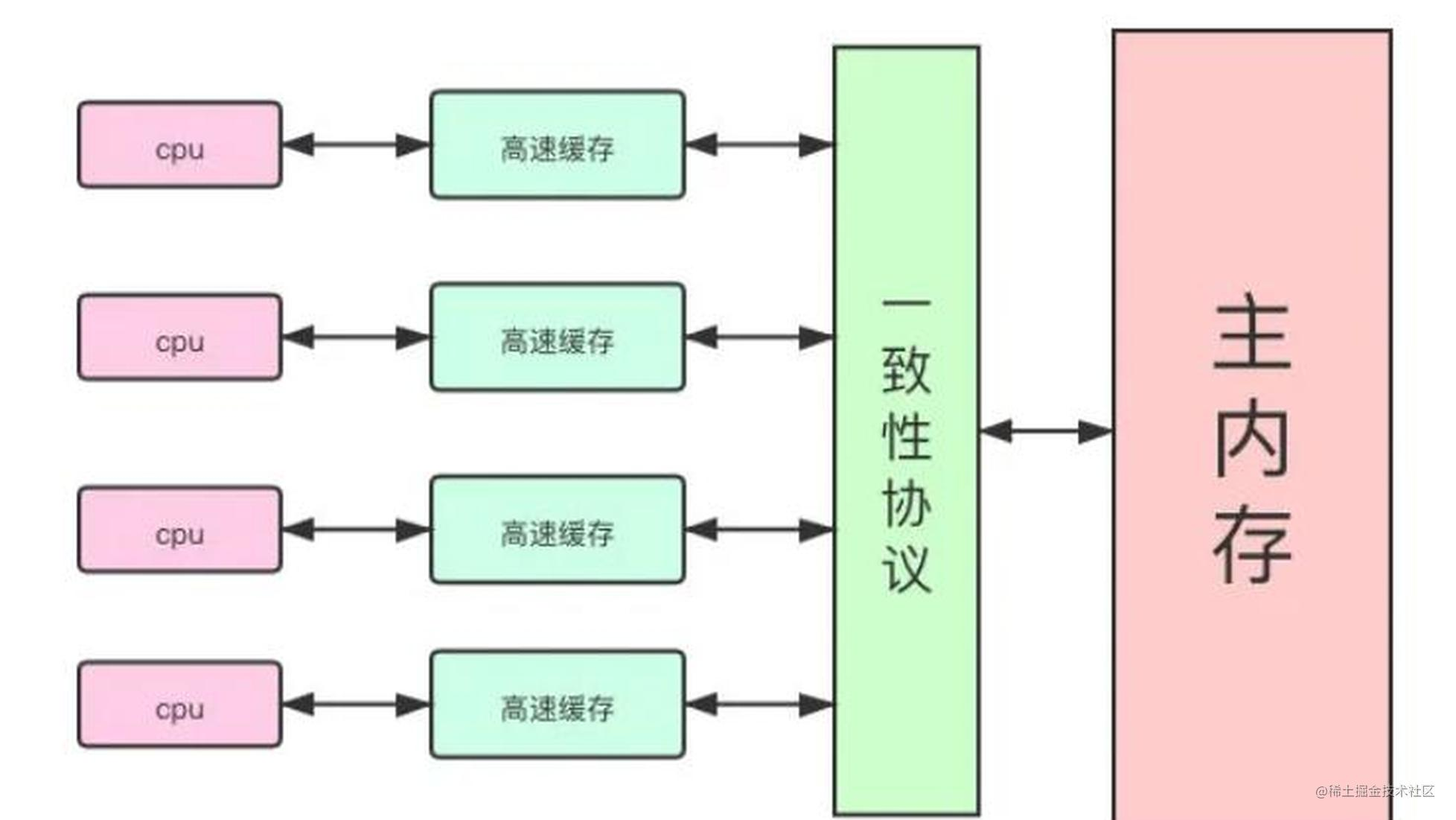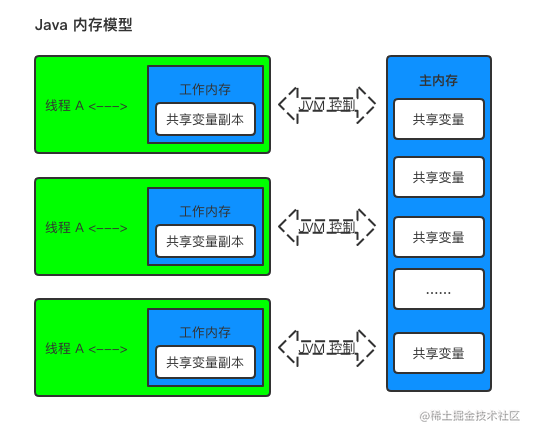学了 JMM 指令重排序，让我明白该如何写单例模式了JMM 是什么？

JMM 是 Java Memory Model 的缩写， Java 内存模型来屏蔽各种硬件和操作系统的内存访问差异，以实现让 Java 程序在各种平台下能够达到一致的内存访问效果。重排序

产生重排序？

/**
* 当m1 和 m2 发生重排序后，先执行x=b，y=a； 在执行a=1，b=1，最后x=0，y=0
*
* @author xx
* @date 2021-08-31
*/
public class JmmTest {

private int a = 0;
private int b = 0;
private int x = 1;
private int y = 1;

public void m1() {
a = 1;
x = b;
}

public void m2() {
b = 1;
y = a;
}

public void test() throws Exception {
CyclicBarrier cyc = new CyclicBarrier(2);
try {
cyc.await();
} catch (Throwable e) {
e.printStackTrace();
}
m1();
}, "A");

try {
cyc.await();
} catch (Throwable e) {
e.printStackTrace();
}
m2();
}, "B");

if (x == 0 && y == 0) {
System.out.println("重排序了。。。。");
}

}

public static void main(String[] args) throws Exception {
for (int i = 0; i < 100000; i++) {
JmmTest jmmTest = new JmmTest();
jmmTest.test();
}
}
}

// 输出结果

final 关键字

final 用于对象应用时，final 使应用恒定不变。一旦引用被初始化指向一个对象，就无法再把它改为指向另一个对象。

final 关键字如何工作？

class FinalFieldExample {
final int x;
int y;
static FinalFieldExample f;

public FinalFieldExample() {
x = 3;
y = 4;
}

static void writer() {
f = new FinalFieldExample();
}

if (f != null) {
int i = f.x;
int j = f.y;
}
}
}

x = 3;
y = 4;
// bad construction - allowing this to escape
global.obj = this;
}

volatile 关键字

volatile 字段是用于在线程之间通信状态的特殊字段。对 volatile 的每次读取都将看到任何线程对该 volatile 的最后一次写入；实际上，程序员将它们指定为字段，因为缓存或重新排序的结果永远不会允许看到“过时”值。禁止编译器和运行时在寄存器中分配它们。它们还必须确保在写入之后，它们会从缓存中刷新到主内存，以便其他线程可以立即看到它们。类似地，在读取易失性字段之前，必须使缓存失效，以便看到的是主存中的值，而不是本地处理器缓存中的值。对易失性变量访问的重新排序也有额外的限制。 ​

class VolatileExample {
int x = 0;
volatile boolean v = false;
public void writer() {
x = 42;
v = true;
}

if (v == true) {
//uses x - guaranteed to see 42.
}
}
}

DCL 单例模式

// double-checked-locking - don't do this!

private static Something instance = null;

public Something getInstance() {
if (instance == null) {
synchronized (this) {
if (instance == null)
instance = new Something();
}
}
return instance;
}

**上面做看上去没有，但是这样写是错误的。 **最明显的原因是初始化 instance 的写入和对 instance 字段的写入可以由编译器或缓存重新排序，这将具有返回看起来是部分构造的东西的效果。结果是我们读取了一个未初始化的对象。还有很多其他原因导致这是错误的，以及为什么对它的算法更正是错误的。 ​

private static class LazySomethingHolder {
public static Something something = new Something();
}

public static Something getInstance() {
return LazySomethingHolder.something;
}